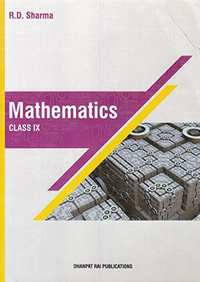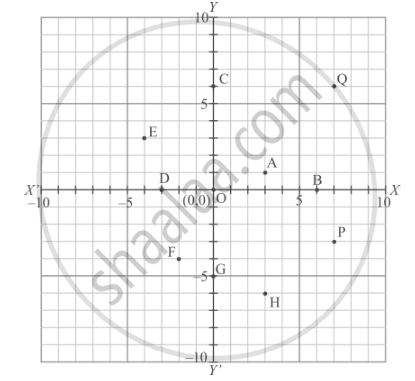Share

# RD Sharma solutions for Mathematics for Class 9 chapter 8 - Co-ordinate Geometry [Latest edition]

Course
Textbook page

#### Chapters## Chapter 8: Co-ordinate Geometry

Ex. 8.1Others

#### RD Sharma solutions for Mathematics for Class 9 Chapter 8 Co-ordinate GeometryExercise 8.1 [Page 6]

Ex. 8.1 | Q 1.01 | Page 6

Plot the following point on the graph paper:

(2,5)

Ex. 8.1 | Q 1.02 | Page 6

Plot the following point on the graph paper:

(4, −3)

Ex. 8.1 | Q 1.03 | Page 6

Plot the following point on the graph paper:

(−5, −7)

Ex. 8.1 | Q 1.04 | Page 6

Plot the following point on the graph paper:

(7, −4)

Ex. 8.1 | Q 1.05 | Page 6

Plot the following point on the graph paper:

(−3, 2)

Ex. 8.1 | Q 1.06 | Page 6

Plot the following point on the graph paper:

(7, 0)

Ex. 8.1 | Q 1.07 | Page 6

Plot the following point on the graph paper:

(−4, 0)

Ex. 8.1 | Q 1.08 | Page 6

Plot the following point on the graph paper:

(0, 7)

Ex. 8.1 | Q 1.09 | Page 6

Plot the following point on the graph paper:

(0, −4)

Ex. 8.1 | Q 1.1 | Page 6

Plot the following point on the graph paper:

(0, 0)

Ex. 8.1 | Q 2 | Page 6

Write the coordinates of each of the following points marked in the graph paper:#### RD Sharma solutions for Mathematics for Class 9 Chapter 8 Co-ordinate Geometry [Page 7]

Q 1 | Page 7

Mark the correct alternative in each of the following:
The point of intersect of the coordinate axes is

• ordinate

• abscissa

•  origin

Q 2 | Page 7

The abscissa and ordinate of the origin are

• (0, 0)

• (1, 0)

• (0, 1)

• (1 , 1)

Q 3 | Page 7

The measure of the angle between the coordinate axes is

• 90°

• 180°

• 360°

Q 4 | Page 7

A point whose abscissa and ordinate are 2 and −5 respectively, lies in

Q 5 | Page 7

Points (−4, 0) and (7, 0) lie

• on x-axis

• y-axis

Q 6 | Page 7

The ordinate of any point on x-axis is

• 0

• 1

• -1

• any number

Q 7 | Page 7

The abscissa of any point on y-axis is

• 0

• 1

• -1

• any number

Q 8 | Page 7

The abscissa of a point is positive in the

Q 9 | Page 7

A point whose abscissa is −3 and ordinate 2 lies in

Q 10 | Page 7

Two points having same abscissae but different ordinate lie on

• x-axis

• y-axis

• a line parallel to y-axis

• a line parallel to x-axis

Q 11 | Page 7

The perpendicular distance of the point P (4, 3) from x-axis is

• 4

• 3

• 5

• none of these

Q 12 | Page 7

The perpendicular distance of the P (4,3)  from y-axis is

• 4

• 3

• 5

• none of these

Q 13 | Page 7

The distance of the point P (4, 3) from the origin is

• 4

• 3

• 5

• 7

Q 14 | Page 7

The area of the triangle formed by the points A(2,0) B(6,0)  and C(4,6) is

•  24 sq. units

• 12 sq. units

• 10 sq. units

• none of these

Q 15 | Page 7

The area of the triangle formed by the points P (0, 1), Q (0, 5) and R (3, 4) is

• 16 sq. units

• 8 sq. units

• 4 sq. units

• 6 sq. units

## Chapter 8: Co-ordinate Geometry

Ex. 8.1Others## RD Sharma solutions for Mathematics for Class 9 chapter 8 - Co-ordinate Geometry

RD Sharma solutions for Mathematics for Class 9 chapter 8 (Co-ordinate Geometry) include all questions with solution and detail explanation. This will clear students doubts about any question and improve application skills while preparing for board exams. The detailed, step-by-step solutions will help you understand the concepts better and clear your confusions, if any. Shaalaa.com has the CBSE Mathematics for Class 9 solutions in a manner that help students grasp basic concepts better and faster.

Further, we at Shaalaa.com provide such solutions so that students can prepare for written exams. RD Sharma textbook solutions can be a core help for self-study and acts as a perfect self-help guidance for students.

Concepts covered in Mathematics for Class 9 chapter 8 Co-ordinate Geometry are Introduction of Coordinate Geometry, Cartesian System, Plotting a Point in the Plane If Its Coordinates Are Given.

Using RD Sharma Class 9 solutions Co-ordinate Geometry exercise by students are an easy way to prepare for the exams, as they involve solutions arranged chapter-wise also page wise. The questions involved in RD Sharma Solutions are important questions that can be asked in the final exam. Maximum students of CBSE Class 9 prefer RD Sharma Textbook Solutions to score more in exam.

Get the free view of chapter 8 Co-ordinate Geometry Class 9 extra questions for Mathematics for Class 9 and can use Shaalaa.com to keep it handy for your exam preparation

S